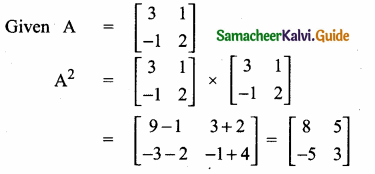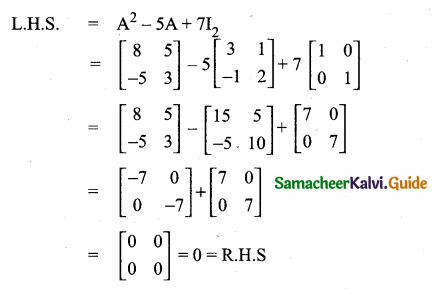Students can download Maths Chapter 3 Algebra Ex 3.18 Questions and Answers, Notes, Samacheer Kalvi 10th Maths Guide Pdf helps you to revise the complete Tamilnadu State Board New Syllabus, helps students complete homework assignments and to score high marks in board exams.

## Tamilnadu Samacheer Kalvi 10th Maths Solutions Chapter 3 Algebra Ex 3.18

Question 1.
Find the order of the product matrix AB if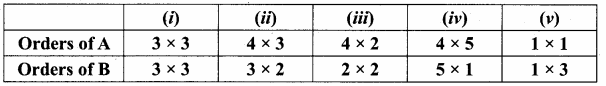Given A = [aij]p×q and B = [aij]q×r
Order of product of AB = p × r
Order of product of BA is not defined. Number columns in r is not equal to the number of rows in P.
∴ Product BA is not defined.Question 2.
A has ‘a’ rows and ‘a + 3 ’ columns. B has ‘6’ rows and ‘17 – b’ columns, and if both products AB and BA exist, find a, b?
Solution:
A has a rows, a + 3 columns.
B has b rows, 17 – b columns
If AB exists a × a + 3
b × 17 – b
a + 3 = 6 ⇒ a – 6 = -3 ………… (1)
If BA exists 6 × 17-6
a × a + 3
17 – 6 = a ⇒ a + 6 = 17 …………. (2)
(1) + (2) ⇒ 2a = 14 ⇒ a = 7
Substitute a = 7 in (1) ⇒ 7 – b = -3 ⇒ b = 10
a = 7, b = 10Question 3.
A has ‘a’ rows and ‘a + 3 ’ columns. B has rows and ‘b’ columns, and if both products AB and BA exist, find a,b?

1. Order of matrix AB = 3 × 3
2. Order of matrix AB = 4 × 2
3. Order of matrix AB = 4 × 2
4. Order of matrix AB = 4 × 1
5. Order of matrix AB = 1 × 3

Question 4.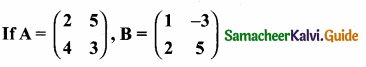find AB, BA and check if AB = BA?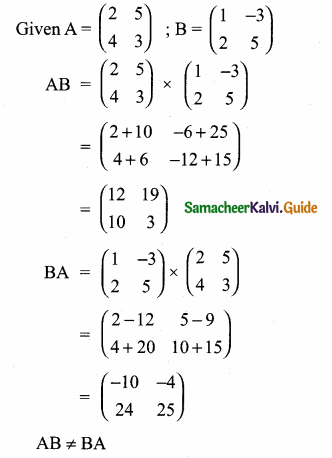Question 5.
Given that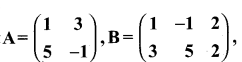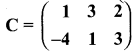verify that A(B + C) = AB + AC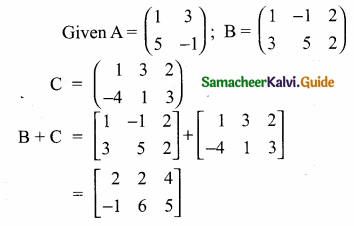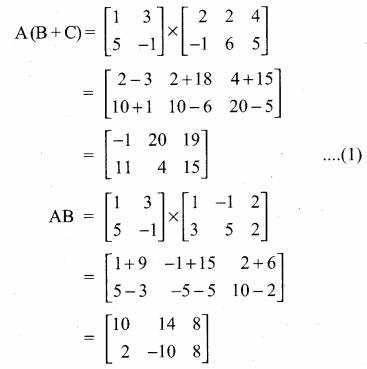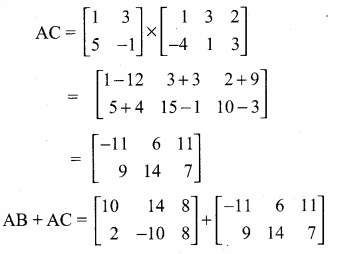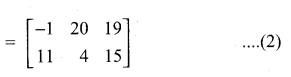From (1) and (2) we get
A (B + C) = AB + ACQuestion 6.
Show that the matrices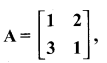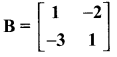satisfy commutative property AB = BA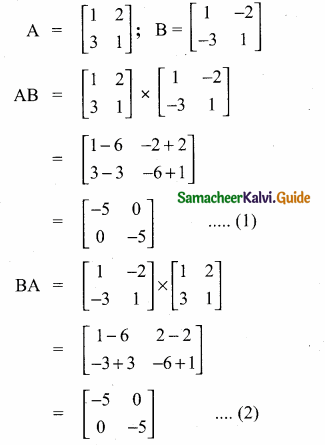From (1) and (2) we get
AB = BA. It satisfy the commutative property.

Question 7.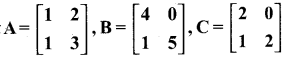Show that (i) A(BC) = (AB)C
(ii) (A-B)C = AC – BC
(iii) (A-B)T = AT – BT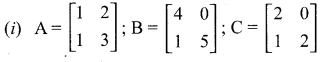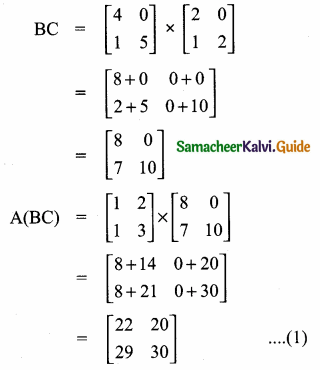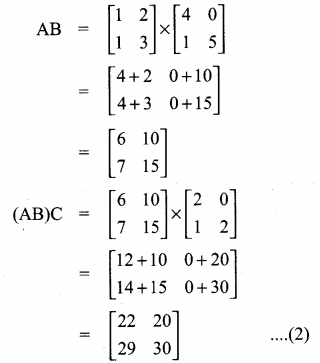From (1) and (2) we get
A(BC) = (AB)C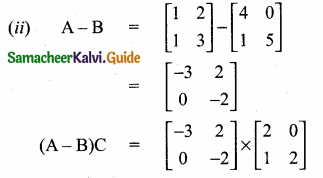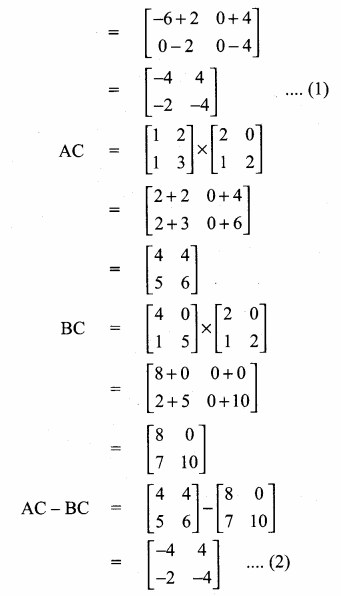From (1) and (2) we get
(A – B) C = AC – BC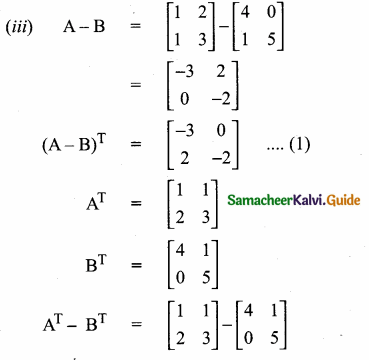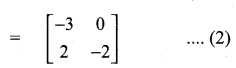From (1) and (2) we get
(A-B)T = AT – BTQuestion 8.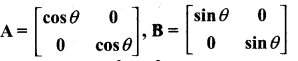then snow that A2 + B2 = I.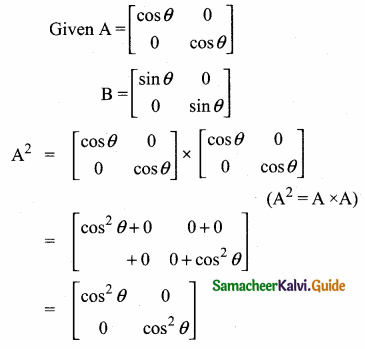Question 9.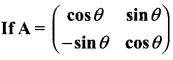prove that AAT = I.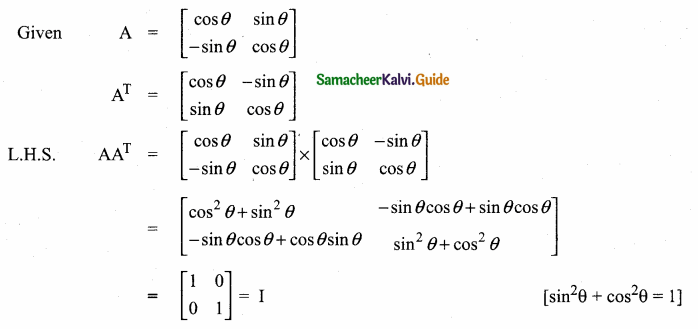AAT = I
∴ L.H.S. = R.H.S.Question 10.
Verify that A2 = I when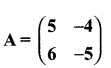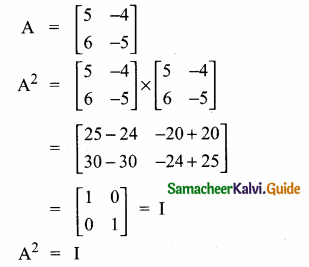∴ L.H.S. = R.H.S.

Question 11.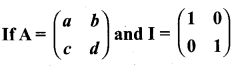show that A2 – (a + d)A = (bc – ad)I2.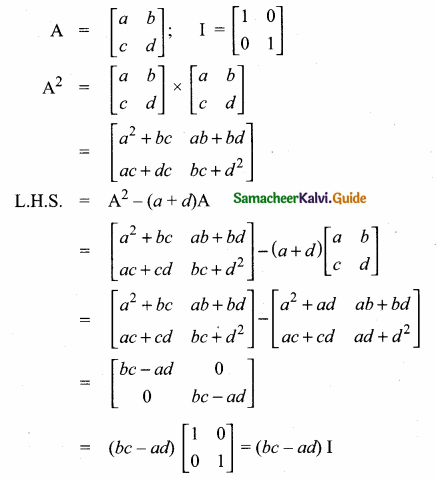L.H.S. = R.H.S.
A2 – (a + d) A = (bc – ad)I2Question 12.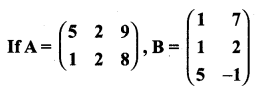verify that (AB)T = BT AT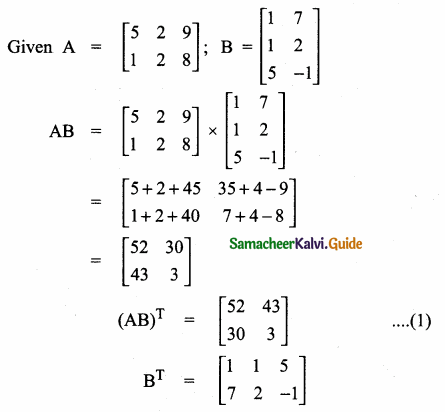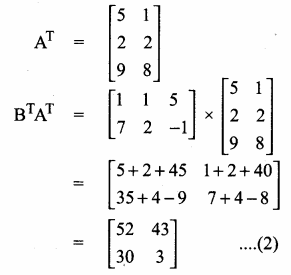From (1) and (2) we get, (AB)T = BT ATQuestion 13.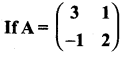show that A2 – 5A + 7I2 = 0